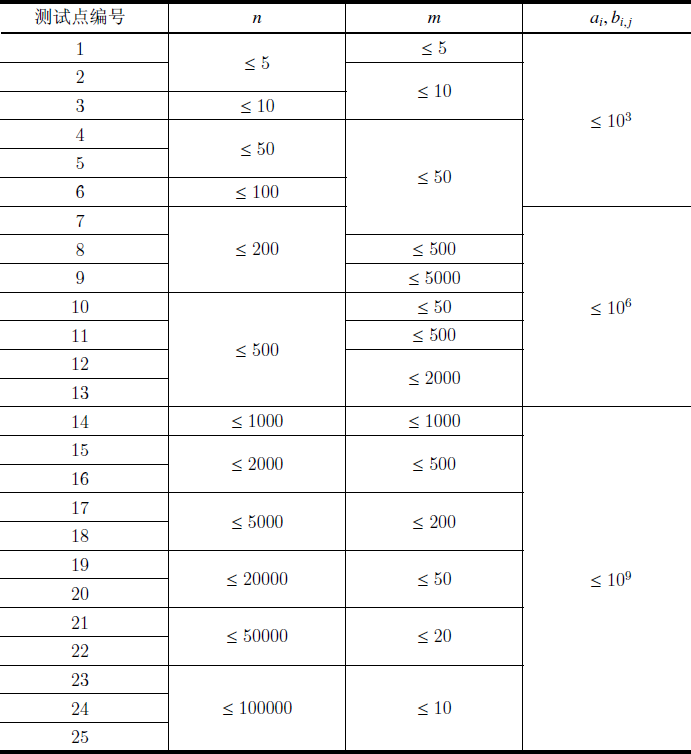# YZOJ P2050 [FJOI2013]圆形游戏

• ### 题目描述

1，选定一个圆 $$A$$，把 $$A$$ 以及所有完全在 $$A$$ 内部的圆都删除；

2，如果在自己回合无法找到可删除的圆，则输掉比赛。

• ### 数据规模与约定

$$100\%$$ 的数据满足 $$T \leq 100$$，$$n \leq m20000$$，$$\left|x\right|, \left|y\right|, r \leq 10^8$$ 。

…

…

# YZOJ P2358 [ZJOI 2012]Naive – Matrix

• ### 数据规模与约定

Source: BZOJ 2658…

# YZOJ P3791 餐馆

• ### 数据规模与约定Source：ARC067 F – Yakiniku